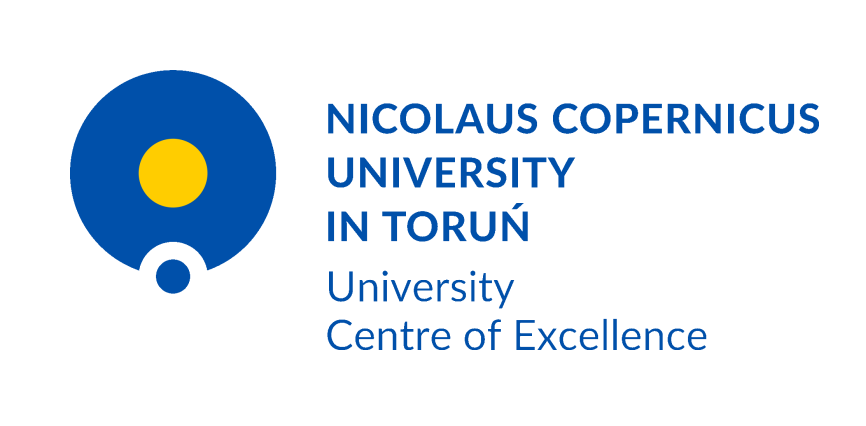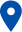### University Centre of Excellence “Dynamics, Mathematical Analysis and Artificial Intelligence”ul. Gagarina 11, 87-100 Toruń
e-mail: damsi@umk.pl

University Centre of Excellence in the research area “Dynamics, mathematical analysis and artificial intelligence”

The thematic scope of the University Centre of Excellence “Dynamics, mathematical analysis and artificial intelligence” includes issues related to dynamical systems and ergodic theory. In addition, the Centre’s work will focus on analytic number theory, computer science, open quantum systems and quantum information. As part of research on artificial intelligence, researchers will focus on brain signal analysis, neurodynamics, and machine learning. The centre specifies four areas of research:

1. Dynamics and ergodic theory:

This research topic continues and develops the main trends of modern dynamics and ergodic theory which rely on the study of relations of both these theories with number theory, combinatorics, geometry and mathematical analysis. In particular, it is worth pointing out the recent significant progress in understanding the relationship between ergodic theory and analytic number theory, indicating numerous connections and possible applications. Toruń dynamists also make a significant contribution to the development of research on this subject.

2. Computer science – formal languages and concurrency:

In computer science, research is conducted in the field of concurrency theory, formal languages, automata, machine learning and data mining. In particular, they concern the theoretical and practical modeling and analysis of concurrent systems (including Petri nets and reaction systems) as well as solving problems with incomplete information, using, among others, methods combining combinatorics, algebra, topology and modal logic.

3. Neuroinformatics and artificial intelligence:

Neuroinformatics is a combination of two important disciplines on the science front: brain research and artificial intelligence. By using machine learning and signal processing methods, new theories and algorithms for brain signal analysis are developed, verifying hypotheses through experiments.

4. Entangled states and dynamics of open quantum systems:

The theory of open quantum systems describes the dynamical evolution of a quantum system interacting with its environment, thus going beyond the standard Schrödinger model of quantum dynamics. It encompasses in articular the elements of quantum information theory and the theory of quantum entanglement, advanced mathematical methods of operator algebras, quantum stochastic processes, non-Markovian dynamics, and random matrix theory.

When studying the mathematical aspects of the above-mentioned main research areas, due to the mathematical tools used, the Centre intends to cooperate with other strong mathematical schools in Toruń: algebra, mathematical analysis and probability theory. In addition, the Centre will cooperate with foreign centers: Aix-Marseille Université in France, Universität Bielefeld in Germany and the University of Zurich in Switzerland.